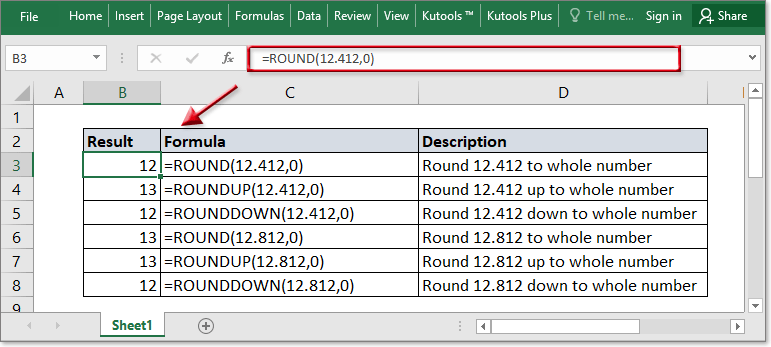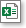Note: The other languages of the website are Google-translated. Back to English

## Convert decimal to whole number in Excel

This tutorial will tell you how to use formulas to convert decimal numbers to whole numbers in Excel.### Round decimal to whole number

Generic formula:

 =ROUND(number,0)

Arguments

 Number: Required, the decimal number you want to convert to a whole number. 0: Required, no decimal digits that you want to retain.

Remarks

The decimal number will be rounded up if the first decimal digit >= 5, or it will be rounded down.

Examples

 Formula Description Result =ROUND(12.722,0)) Round 12.722 to whole number 13 =ROUND(12.222,0) Round 12.222 to whole number 11 =ROUND(12.5,0) Round 12.5 to whole number 13

### Round decimal up to whole number

Generic formula:

 =ROUNDUP(number,0)

Arguments

 Number: the decimal number you want to round up to a whole number. 0: Required, no decimal digits that you want to retain.

Remarks

The ROUNDUP function will round decimal numbers up to nearest whole number.

Examples

 Formula Description Result =ROUNDUP(1.03,0) Round 1.03 up to nearest whole number 2 =ROUNDUP(1.92,0) Round 1.92 up to nearest whole number 2

### Round decimal down to whole number

Generic formula:

 =ROUNDDOWN(number,0)

Arguments

 Number: Required, the decimal number you want to round down to a whole number. 0: Required, no decimal digits that you want to retain.

Remarks

The ROUNDDOWN function will round decimal numbers down to nearest whole number.

Examples

 Formula Description Result =ROUNDDOWN(123.234,0) Round 123.234 down to whole number 123 =ROUNDDOWN(123.662,0) Round 123.662 down to whole number 123

### Sample FileClick to download sample file

#### Relative Functions

• Excel DECIMAL function
The DECIMAL function converts a text representation of a number in a base into its equivalent decimal number. Take a instance, 11 is a text representation of 3 in base 2, using the formula =DECIMAL(11,2) convert 11 into decimal number 3.

### The Best Office Productivity Tools

#### Kutools for Excel - Helps You To Stand Out From Crowd

Would you like to complete your daily work quickly and perfectly? Kutools for Excel brings 300 powerful advanced features (Combine workbooks, sum by color, split cell contents, convert date, and so on...) and save 80% time for you.

• Designed for 1500 work scenarios, helps you solve 80% Excel problems.
• Reduce thousands of keyboard and mouse clicks every day, relieve your tired eyes and hands.
• Become an Excel expert in 3 minutes. No longer need to remember any painful formulas and VBA codes.
• 30-day unlimited free trial. 60-day money back guarantee. Free upgrade and support for 2 years.#### Office Tab - Enable Tabbed Reading and Editing in Microsoft Office (include Excel)

• One second to switch between dozens of open documents!
• Reduce hundreds of mouse clicks for you every day, say goodbye to mouse hand.
• Increases your productivity by 50% when viewing and editing multiple documents.
• Brings Efficient Tabs to Office (include Excel), Just Like Chrome, Firefox, And New Internet Explorer.Rated 5 out of 5 · 1 ratings
This comment was minimized by the moderator on the site
MY 0 DOESNT APPEAR IN THE FIRST ROW . EXAMPLE IF I TYPE 0158691 THE 0 DOESNT APPEAR
This comment was minimized by the moderator on the site
Hi，Pamela Newton, if you want to keep the leading zeros while you are typing, this tutorial https://www.extendoffice.com/documents/excel/1778-excel-delete-keep-leading-zeros.html lists some methods may help you
This comment was minimized by the moderator on the site
How to convert 0.2677 into 2,677?
This comment was minimized by the moderator on the site
0.2677*10000
This comment was minimized by the moderator on the site
0.00006944 as a whole number
This comment was minimized by the moderator on the site
2885/1000=2.885.I WANT TO APPEAR ONLY FULL DIGIT ONLY (2),ANYBODY TO EXPLAIN?
This comment was minimized by the moderator on the site
You can use INT() function
This comment was minimized by the moderator on the site
how to convert 570 to 600
This comment was minimized by the moderator on the site
A1=570，IF(RIGHT(A1,2)>=5,(RIGHT(A1,3)+1)*100,RIGHT(A1,3)*100)

...

This comment was minimized by the moderator on the site
use =ROUND(A1,-1)
OR
=ROUND(A1,-2)
Rated 5 out of 5
This comment was minimized by the moderator on the site
how to convert 8.25 lakhs to 825000
This comment was minimized by the moderator on the site
Hi, rashmi, if only numbers in the cell, using the numbers to multiply 100000 such as =8.25*100000; if there is numbers and texts in a cell, using text to columns feature to split the text and numbers into two columns, then multiply 1000000.
Please let me know if work for you.
This comment was minimized by the moderator on the site
Bonjour ! je souhaite revenir à la cellule normale qui m'écrirait 12 et non 0,00012 comme c'est le cas en ce moment dans ma machine : je ne sais plus qu'est-ce que j'ai touché pour y parvenir. merci.
This comment was minimized by the moderator on the site
Merhaba
Formül de bir sayının %70 ni aldığımda 73,5 çıkıyor bunu da 7 ile çarptığımda ise 514.5 çıkıyor. Ama hesaplama da ben 74*7 yaptığımda 518 olmasını sağlamak isityorum. Bu sayı tam olarak nasıl çarptırım excelde ...Teşekkür ederim.
This comment was minimized by the moderator on the site
Hi, using ROUNDUP function to round 73.5 to 74 and then multiply 7.
=ROUNDUP(73.5,0)*7
There are no comments posted here yet